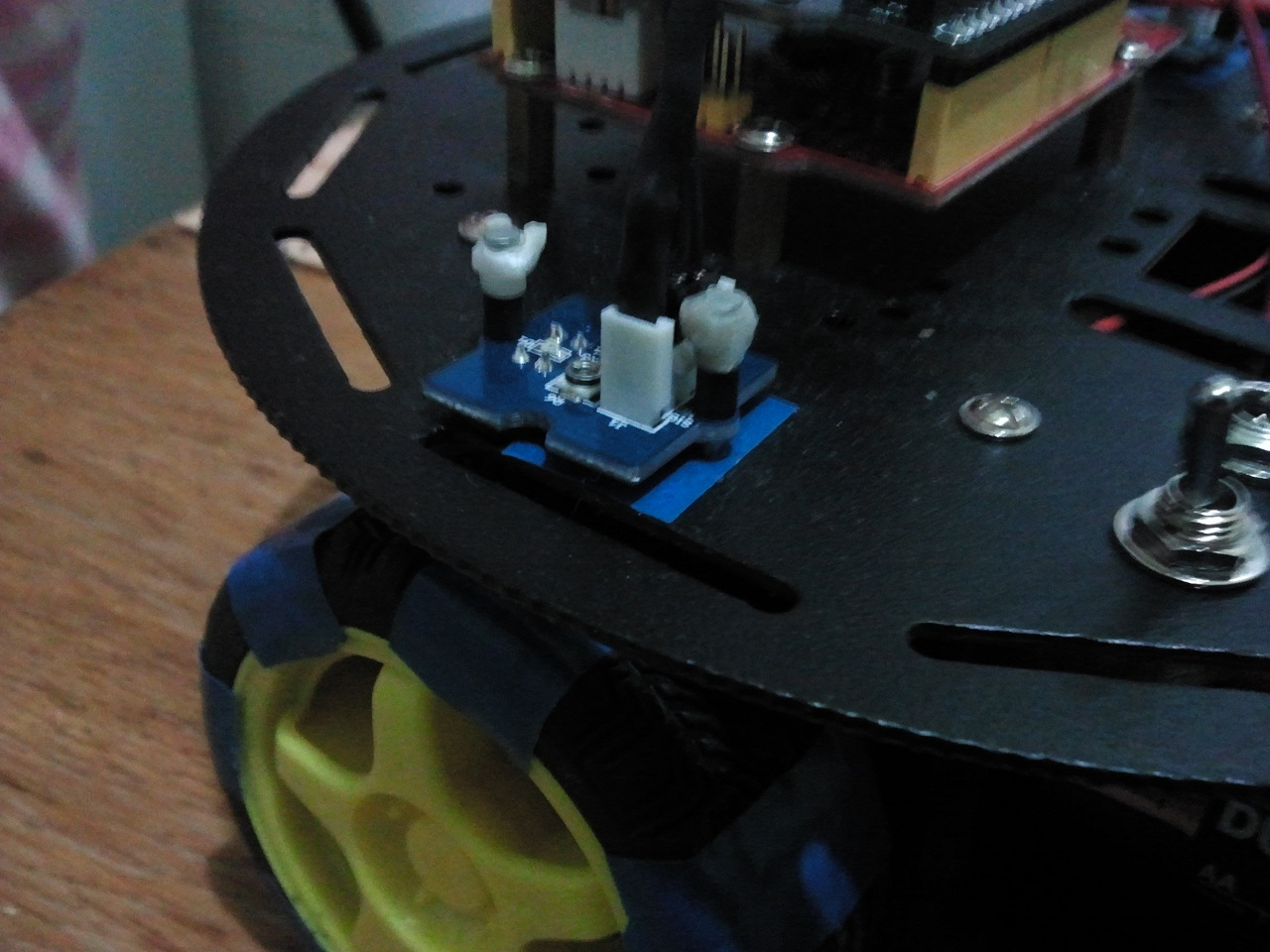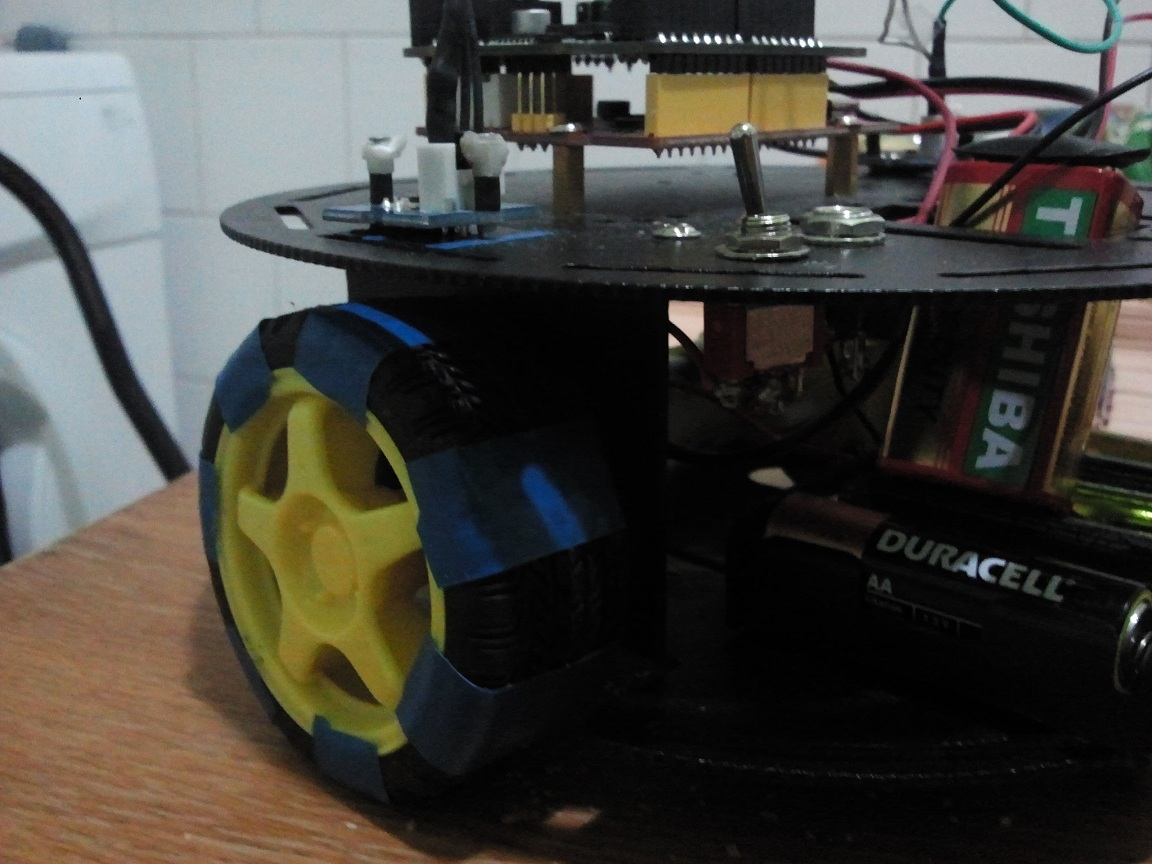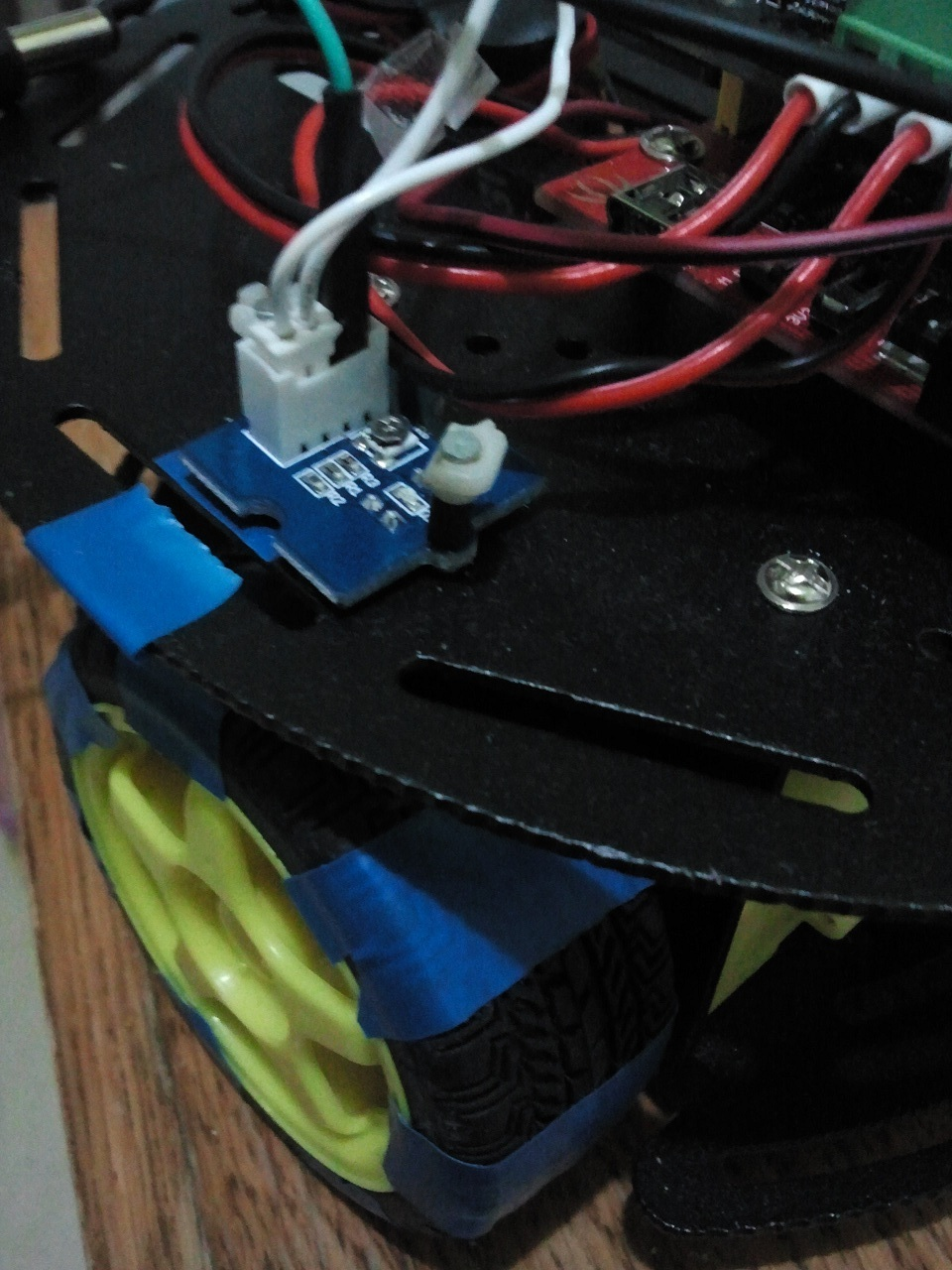# Seeeduino + 2A Motor Shield For Arduino + Grove Line sensor

Hi,
I am trying to calibrate 2 motors with 2 grove line sensors using interrupts, trying to count the number of white stripes from the wheel.

Demo code:

``````//Arduino PWM Speed Control：
int E1 = 6;
int M1 = 7;
int E2 = 5;
int M2 = 4;
int leftSensor = 0; // PIN 2 on arduino
volatile int count_left = 0;
void setup()
{
pinMode(M1, OUTPUT);
pinMode(M2, OUTPUT);
attachInterrupt(leftSensor, CountLeft, RISING );
Serial.begin(9600);
}

void loop()
{
digitalWrite(M1,HIGH);
digitalWrite(M2, HIGH);
analogWrite(E1, 80);   //PWM Speed Control
analogWrite(E2, 80);   //PWM Speed Control
Serial.println(count_left);
}
void CountLeft()
{
count_left++;
}``````

In this case when the count_left variable is very high (for example the wheel is rotating 2 white strips => count_left = 80…100) , but when i move the wheel manually (deactivating the motors) the count_left is correct. 1 white stripe => count_left=1; 2 white stripes = count_left=2;

My question is why when i am using the motors the count_left is going crazy?

Dont use serial commands inside a interrupt routine!

Hi,For left sensor i use port 2 and for the right senzor i use the port 3.
Is it possible that using the same 5v port and gnd from motor shield to affect the sensors?

Use white tape on your wheels. The Line Sensor need a high contrast.
5V from Motor Driver must be ok if your power source (battery?) id strong enougth.

The tape is not the problem because the sensors are working fine ( i used the adjustable resistor to set a suitable reference voltage to detect blue tape).

When moving the wheel with the hand the sensor detects the blue(tape) and black.
I can`t understand why the output(count) is so different with the motor shield.

Output:
Moving the wheel manually 2 black lines : count_left=2;
Moving the wheel with the motor shield 2 black lines : count_left=for example 70 (a very high value).
Why?
I will try to upload a video with my tests to see exactly what is happening.
Thanks,